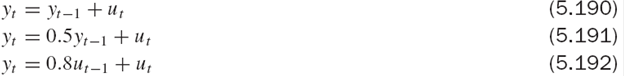### Create an Account

Home / Questions / What are the differences between autoregressive and moving average models Why might ARMA m...

# What are the differences between autoregressive and moving average models Why might ARMA models be considered particularly useful for financial time series

1. What are the differences between autoregressive and moving average models?

2. Why might ARMA models be considered particularly useful for financial time series? Explain, without using any equations or mathematical notation, the difference between AR, MA and ARMA processes.

3. Consider the following three models that a researcher suggests might be a reasonable model of stock market prices(a) What classes of models are these examples of?

(b) What would the autocorrelation function for each of these processes look like? (You do not need to calculate the acf, simply consider what shape it might have given the class of model from

which it is drawn.)

(c) Which model is more likely to represent stock market prices from a theoretical perspective, and why? If any of the three models truly represented the way stock market prices move, which could potentially be used to make money by forecasting future values of the series?

(d) By making a series of successive substitutions or from your knowledge of the behaviour of these types of processes, consider the extent of persistence of shocks in the series in each case.

Apr 29 2020 View more View LessSubscribe To Get Solution